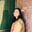Trusted answers to developer questions
Trusted Answers to Developer Questions

Related Tags

numpy
python

# What is numpy.isfinite() in Python?Umme Ammara

Grokking Modern System Design Interview for Engineers & Managers

Ace your System Design Interview and take your career to the next level. Learn to handle the design of applications like Netflix, Quora, Facebook, Uber, and many more in a 45-min interview. Learn the RESHADED framework for architecting web-scale applications by determining requirements, constraints, and assumptions before diving into a step-by-step design process.Python’s numpy.isfinite() tests if an element is finite or not. It tests an array element-wise and returns a Boolean array as the output.

## Syntax

numpy.isfinite() is declared as follows:

numpy.isfinite(x, /, out=None, *, where=True, casting='same_kind', order='K', dtype=None, subok=True[, signature, extobj]) = <ufunc 'isfinite'>


In the syntax above, x is the non-optional parameter and the rest are optional parameters.

A universal function (ufunc) is a function that operates on ndarrays in an element-by-element fashion. The isfinite() method is a universal function.

## Parameters

The numpy.isfinite() method takes the following compulsory parameter:

• x [array-like] - input array.

The numpy.isfinite() method takes the following optional parameters:

 Parameter Description out represents the location into which the output of the method is stored. where True value indicates that a universal function should be calculated at this position. casting controls the type of datacasting that should occur. The same_kind option indicates that safe casting or casting within the same kind should take place. order controls the memory layout order of the output function. The option K means reading the elements in the order they occur in memory. dtype represents the desired data type of the array. subok

## Return value

numpy.isfinite() returns Boolean values True or False depending on the following:

• It returns False if x is positive infinity, negative infinity or NaN.

• It returns True in all other cases.

If x is scalar, the return type is also scalar.

## Examples

The example below shows the use of numpy.isfinite() on the elements a and b:

import numpy as npa = 23b = np.nanprint (np.isfinite(a))print (np.isfinite(b))

The following example shows the use of numpy.isfinite() on the array arr1:

import numpy as np

arr1 = np.array([2, np.inf, 0, 10000000000000])
print (np.isfinite(arr1))

The following example shows the use of numpy.isfinite() on the array arr2:

import numpy as nparr2 = np.array([[1, 2, 3], [np.inf, np.nan, -np.inf]])print (np.isfinite(arr2))

RELATED TAGS

numpy
python

CONTRIBUTORUmme Ammara